Engineering Mechanics - PKRB: Impulse and Momentum

Exercise :: PKRB: Impulse and Momentum - General Questions

• PKRB: Impulse and Momentum - General Questions
6.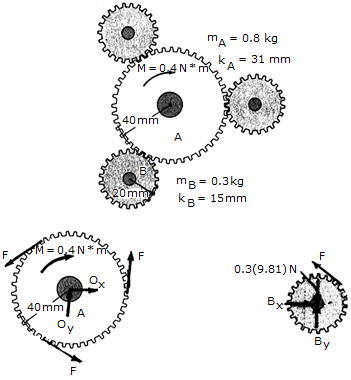A constant torque or twist of M = 0.4N • m is applied to the center gear A. If the system starts from rest, determine the angular velocity of each of the three (equal) smaller gears in 3 s. The smaller gears (B) are pinned at their centers, and the mass and centroidal radii of gyration of the gears are given in the figure.

 A.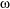B = 1975 rad/s B.B = 5830 rad/s C.B = 1520 rad/s D.B = 1022 rad/s

Explanation:

No answer description available for this question. Let us discuss.

7.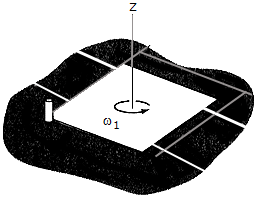The square plate, where a = 0.75 ft, has a weight of 4 lb and is rotating on the smooth surface with a constant angular velocity of1 = 10 rad/s. Determine the new angular velocity of the plate just after its corner strikes the peg P and the plate to rotate about P without rebounding.

 A.2 = 6.67 rad/s B.2 = 2.50 rad/s C.2 = 10.00 rad/s D.2 = 5.00 rad/s

Explanation:

No answer description available for this question. Let us discuss.

8.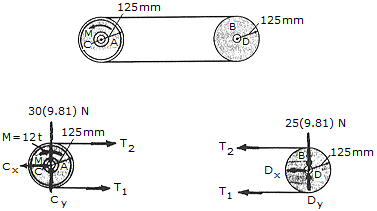The flywheel A has a mass of 30 kg and a radius of gyration of kc = 95 mm. Disk B has a mass of 25 kg, is pinned at D, and is coupled to the flywheel using a belt which is subjected to a tension such that it does not slip at its contacting surfaces. If a motor supplies a counter-clockwise torque or twist to the flywheel, having a magnitude of M = (12t) N • m, where t is measured in seconds, determine the angular velocity of the disk 3 s after the motor is turned on. Initially, the flywheel is at rest.

 A.= 232 rad/s B.= 77.2 rad/s C.= 115.9 rad/s D.= 276 rad/s

Explanation:

No answer description available for this question. Let us discuss.

9.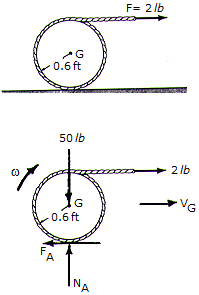A cord of negligible mass is wrapped around the outer surface of the 50-lb cylinder and its end is subjected to a constant horizontal force of P = 2 lb. If the cylinder rolls without slipping at A, determine its angular velocity in 4 s starting from rest. Neglect the thickness of the cord.

 A.= 34.3 rad/s B.= 17.17 rad/s C.= 8.59 rad/s D.= 11.45 rad/s

Explanation:

No answer description available for this question. Let us discuss.

10.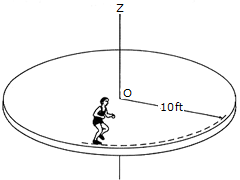A horizontal circular platform has a weight of 300 lb and a radius of gyration about the z axis passing through its center O of kz = 8 ft. The platform is free to rotate about the z axis and is initially at rest. A man, having a weight of 150 lb, begins to run along the edge in a circular path of radius 10 ft. If he has a speed of 4 ft/s and maintains this speed relative to the platform, compute the angular velocity of the platform.

 A.= 0.313 rad/s B.= 0.1754 rad/s C.= 0.225 rad/s D.= 1.429 rad/s